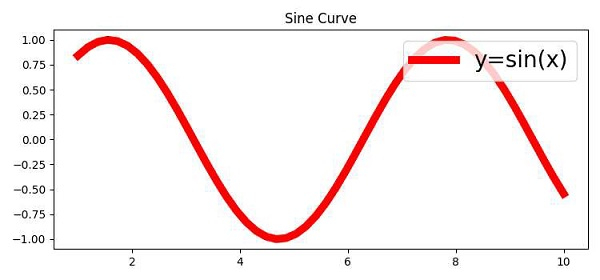# How to set the font size of Matplotlib axis Legend?

To set the font size of matplotlib axis legend, we can take the following steps −

• Create the points for x and y using numpy.

• Plot x and y using the plot() method with label y=sin(x).

• Title the plot using the title() method.

• To set the fontsize, we can override rcParams legend fontsize by value 20.

• Use the legend() method, and fit the legend at the top-right position.

• To display the figure, use the show() method.

## Example

import numpy as np
from matplotlib import pyplot as plt
import matplotlib
plt.rcParams["figure.figsize"] = [7.50, 3.50]
plt.rcParams["figure.autolayout"] = True
x = np.linspace(1, 10, 50)
y = np.sin(x)
plt.plot(x, y, c="red", lw=7, label="y=sin(x)")
plt.title("Sine Curve")
matplotlib.rcParams['legend.fontsize'] = 20
plt.legend(loc=1)
plt.show()

## Output